# Exploring the Panama Papers Network¶

Amsterdam Pydata Meetup

2016-12-08

Iain Barr

## Overview¶

• What are the Panama Papers
• Why am I talking about Exploratory Data Analysis
• Graphs
• Exploring Data

This presentation is based on the work completed during a hackathon run by transparency international in May 2016.

The original write-up is here

This presentation is online at www.degeneratestate.org/static/presentations/pppd2016.html

# The Panama Papers: What?¶

In 2016, the International Consortium of Investigative Journalists(ICIJ) published details of the panama papers. They covered leaked documents from the Panamanian law firm Mossack Fonseca, detailing their business dealings.

• 11 million documents
• 2.6 Terabytes of data
• Largest leak in histroy

# The Panama Papers: Why?¶

“Previously, we thought that the offshore world was a shadowy, but minor, part of our economic system. What we learned from the Panama Papers is that it is the economic system.”

Quote from Panama: the hidden trillions

"Ninety-five per cent of our work coincidentally consists in selling vehicles to avoid taxes."

leaked memorandum from a partner of Mossack Fonseca

# The Panama Papers: Obligitory Disclaimer¶

Morality aside, there are perfectly legal reasons to have an offshore account.

I want to emphasis that:

I am not accusing any individuals or companies that appear in this presentation or in the original dataset of wrong doing.

# Exploratory Data Analysis¶

"Procedures for analyzing data, techniques for interpreting the results of such procedures, ways of planning the gathering of data to make its analysis easier, more precise or more accurate, and all the machinery and results of (mathematical) statistics which apply to analyzing data." - John Tukey

# Graphs¶

Formally, a graph is defined by the ordered pair $G = (V,E)$, where:

• $V$ is a set of vertices
• $E$ is a set of pairs of verticies

Edges can be directed or undirected.

# Graphs in Python¶

We will be using the library NetworkX to explore the data.

NetworkX keeps the graph in memory. For this dataset this isn't an issues, however it may become impractical for larger datasets. Similar approachs to those presented here should work with distributed graph representations such as GraphX.

# Graphs in Python¶

In :
import networkx as nx

# Create a simple undirected graph
g = nx.Graph()

In :
# and plot it
import matplotlib.pyplot as plt
import seaborn as sns # this isn't actually required, but it makes our plots look nice
%matplotlib inline

nx.draw_networkx(g)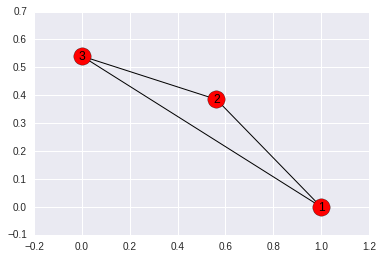# The Data¶

The data released wasn't the raw data, but preprocessed data in the format of a directed graph. The nodes represent things, and the edge represent relationships between things.

Nodes

• entities
• intermediates
• officers

Edges

• intermediary of
• shareholder of
• Records & Registers of
• etc
In :
# Loading the data into pandas for easy processing

ents["name"] = ents.name.apply(normalise)

inter["name"] = inter.name.apply(normalise)

offi["name"] = offi.name.apply(normalise)


In :
# With the nodes and edges in pandas, we can quickly explore various properties
# such as looking at the different types of nodes
edges.rel_type.value_counts()[:20].plot(kind="bar", figsize=(20,5))
plt.title("Types of relationships encoded in edges", fontsize=20)
plt.xticks(fontsize=20);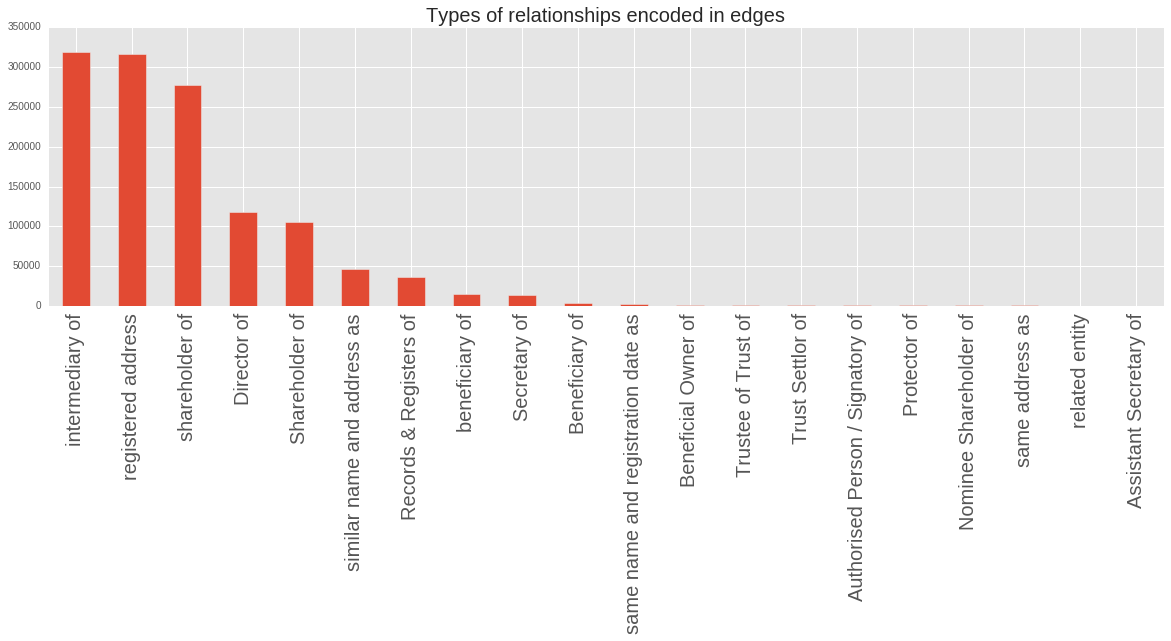In :
# as another example, let's look the top 20 countries for each of the node types

plt.xticks(fontsize=20);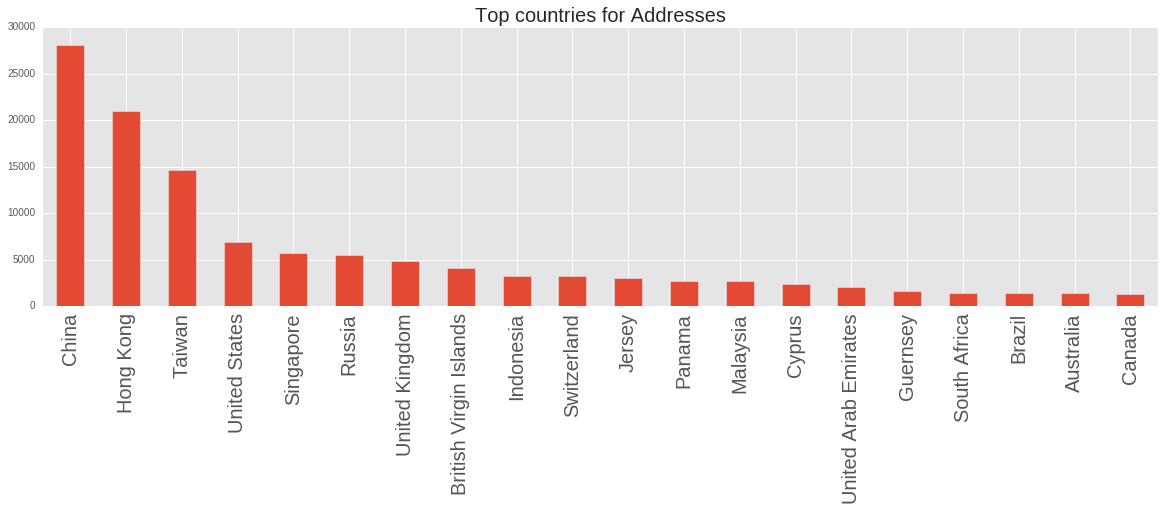In :
ents.countries.value_counts()[:20].plot(kind="bar", figsize=(20,5))
plt.title("Top countries for Entities", fontsize=20)
plt.xticks(fontsize=20);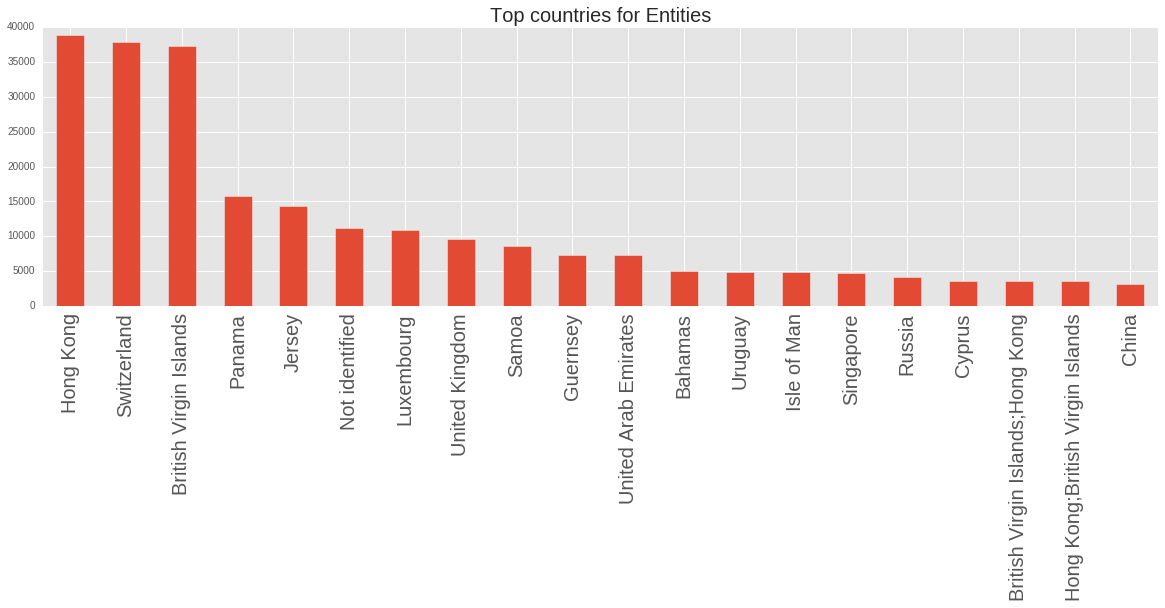In :
inter.countries.value_counts()[:20].plot(kind="bar", figsize=(20,5))
plt.title("Top countries for Intermediates", fontsize=20)
plt.xticks(fontsize=20);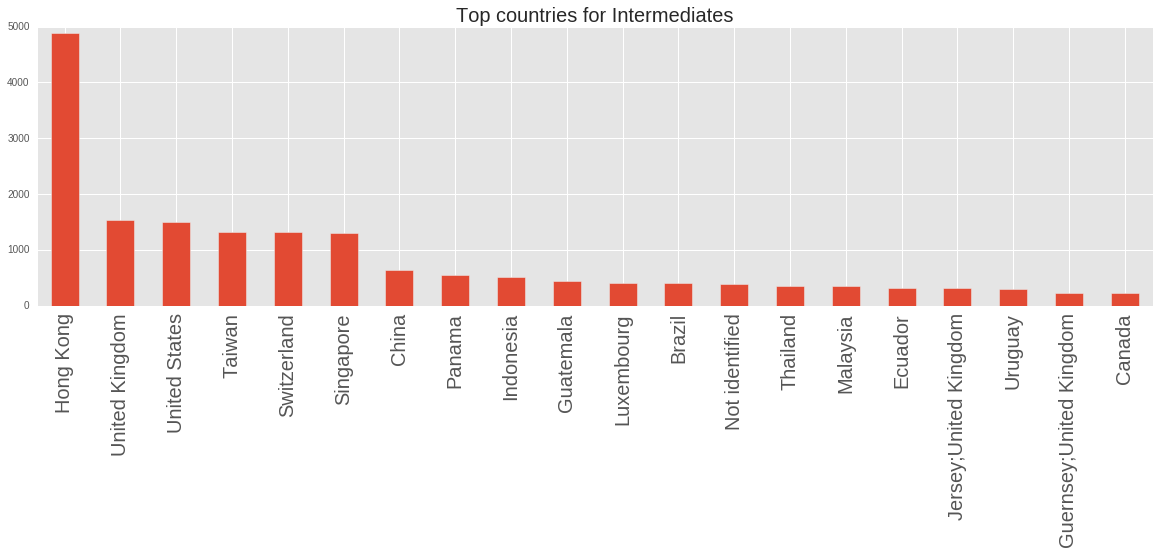In :
offi.countries.value_counts()[:20].plot(kind="bar", figsize=(20,5))
plt.title("Top countries for Officers", fontsize=20)
plt.xticks(fontsize=20);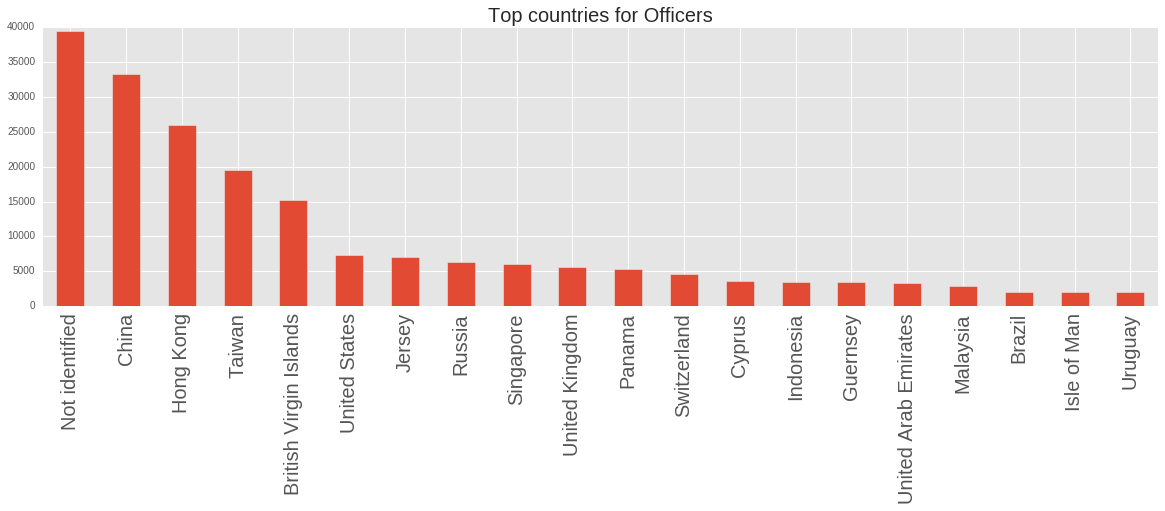# Working with the Graph¶

In :
# create graph

G = nx.DiGraph()

for n,row in ents.iterrows():

for n,row in inter.iterrows():

for n,row in offi.iterrows():

for n,row in edges.iterrows():

In :
print("Number of nodes: {}".format(G.number_of_nodes()))
print("Number of edges: {}".format(G.number_of_edges()))

# Merge similar nodes
merge_similar_names(G)
print("After Merge")

print("Number of nodes: {}".format(G.number_of_nodes()))
print("Number of edges: {}".format(G.number_of_edges()))

Number of nodes: 838295
Number of edges: 1212945
After Merge
Number of nodes: 813423
Number of edges: 1133028


We can now look at whether the graph we are looking at is fully connected or not. NetworkX makes this easy.

In :
# get all connected subgraphs
subgraphs = [g for g in nx.connected_component_subgraphs(G.to_undirected())]

# sort by number of nodes in each
subgraphs = sorted(subgraphs, key=lambda x: x.number_of_nodes(), reverse=True)

# take a look
print([s.number_of_nodes() for s in subgraphs[:10]])

[708807, 728, 644, 597, 521, 409, 398, 378, 378, 372]

In :
plot_graph(subgraphs, figsize=(12,12))